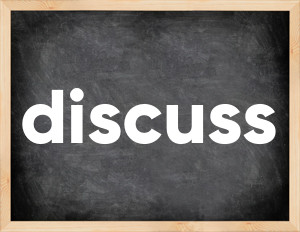# Discuss past tenseThe English verb 'discuss' is pronounced as [dɪˈskʌs].
Related to: regular verbs.
3 forms of verb discuss: Infinitive (discuss), Past Simple - (discussed), Past Participle - (discussed).

## Here are the past tense forms of the verb discuss

👉 Forms of verb discuss in future and past simple and past participle.
❓ What is the past tense of discuss.

## Discuss: Past, Present, and Participle Forms

Base Form Past Simple Past Participle
discuss [dɪˈskʌs]

discussed [dɪˈskʌst]

discussed [dɪˈskʌst]

## What are the 2nd and 3rd forms of the verb discuss?

🎓 What are the past simple, future simple, present perfect, past perfect, and future perfect forms of the base form (infinitive) 'discuss'?

### Learn the three forms of the English verb 'discuss'

• the first form (V1) is 'discuss' used in present simple and future simple tenses.
• the second form (V2) is 'discussed' used in past simple tense.
• the third form (V3) is 'discussed' used in present perfect and past perfect tenses.

## What are the past tense and past participle of discuss?

The past tense and past participle of discuss are: discuss in past simple is discussed, and past participle is discussed.

### What is the past tense of discuss?

The past tense of the verb "discuss" is "discussed", and the past participle is "discussed".

### Verb Tenses

Past simple — discuss in past simple discussed (V2).
Future simple — discuss in future simple is discuss (will + V1).
Present Perfect — discuss in present perfect tense is discussed (have/has + V3).
Past Perfect — discuss in past perfect tense is discussed (had + V3).

### discuss regular or irregular verb?

👉 Is 'discuss' a regular or irregular verb? The verb 'discuss' is regular verb.

## Examples of Verb discuss in Sentences

•   So they can properly discuss the arrangements (Present Simple)
•   Experts discussed an earlier version at a meeting in june (Past Simple)
•   The Technical Sub-group has discussed the first project (Present Perfect)
•   There is nothing to discuss here (Present Simple)
•   There are certain issues I can't discuss (Present Simple)
•   We should discuss this in private (Present Simple)
•   We discussed that before your parents moved in with us (Past Simple)
•   We will discuss it at home, Mathew (Future Simple)
•   It's possible they discussed me with the detectives (Past Simple)
•   It have been discussed in the previous chapters of the book (Past Simple)

Along with discuss, words are popular invent and mow.

Verbs by letter: , , , , , , , , , , , , , , , , , , , , , , , , .